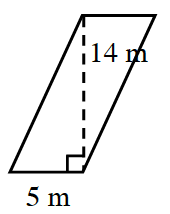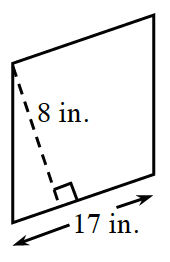### Home > CC1MN > Chapter 6 > Lesson 6.3.3 > Problem6-91

6-91.

Find the area of each parallelogram below. Show all of your work. Use the Math Notes box in this lesson if you need help.

If you were to cut the figure along the dotted line, can you rearrange the shape to form a rectangle?

1.The area of the parallelogram is equal to the product of its base ($5$ m) and its height ($14$ m).

$(5 \text{ m})(14 \text{ m}) = 70 \text{ m}^2$

1.If you rotate the figure in part (b), you will see that the base is $17$ in. and the height is $8$ in.